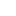v2.28How to obtain object quantities

# Overview

A quantity is a derived measure of a model object's physical property. To get quantities of an object use the IQuantityContainer interface, available via the IModelObject::GetQuantities() and ILayer::GetQuantities() methods. A quantity can be obtained via IQuantityContainer::GetQuantity(). To check if a quantity is available for an object use the IQuantityContainer::HasQuantity() method.

# How to obtain the quantities

The code below demonstrates how to obtain the net volume quantity from an arbitrary model object. The collection of model objects can be received from IModel interface.

## C++

void obtainQuantityContainer(Renga::IModelObjectPtr pModelObject)
{
Renga::IQuantityContainerPtr pQuantityContainer = pModelObject->GetQuantities();
Renga::IQuantityPtr pQuantity = pQuantityContainer->Get(Renga::QuantityIds::NetVolume);
if (pQuantity)
{
if (pQuantity->HasValue == VARIANT_TRUE)
{
const double volume = pQuantity->AsVolume(Renga::VolumeUnit_Meters3);
// TODO: Some actions based on the volume.
}
else
{
// The object does support the NetVolume quantity, but the quantity value could not be calculated.
}
}
else
{
// The object does not support the NetVolume quantity.
}
}

## C#

public void obtainQuantityContainer(Renga.IModelObject modelObject)
{
Renga.IQuantityContainer quantityContainer = modelObject.GetQuantities();
Renga.IQuantity quantity = quantityContainer.Get(Renga.QuantityIds.NetVolume);
if (quantity != null)
{
if (quantity.Type == Renga.QuantityType.QuantityType_Volume)
{
double volume = quantity.AsVolume(Renga.VolumeUnit.VolumeUnit_Meters3);
// TODO: Some actions based on the volume.
}
else
{
// The object does support the NetVolume quantity, but the quantity value could not be calculated.
}
}
else
{
// The object does not support the NetVolume quantity.
}
}